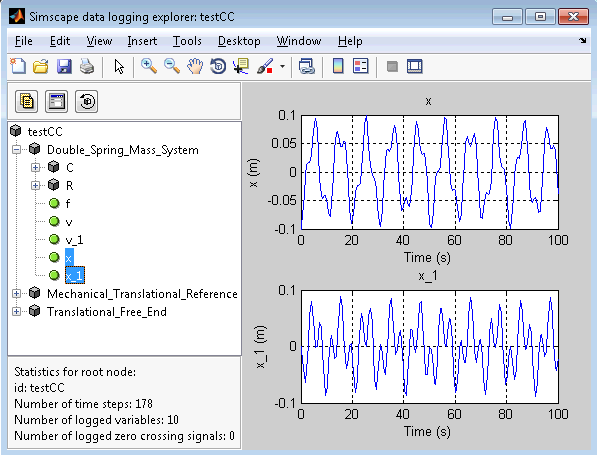I am recently visited the MathWorks Japan office and learned about interesting work done by my colleague Hitoshi Takeshita.In his MATLAB Central submission Euler–Lagrange equation, Hitoshi shows how the Symbolic Math Toolbox can be used to easily obtain the equations of motion of a system by simply defining the energies involved. Once the equations of motion are obtained, he then uses the code generation capability of the Symbolic Math Toolbox to create a Simscape component and simulate the system in Simulink.

I had never used this workflow before, but it can be very useful in applications like plant modeling and for engineering students learning systems dynamics.

Let's look at an example using a double mass-spring system: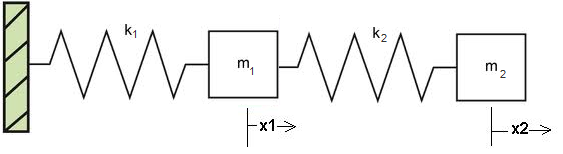Modeling a dynamic system in MuPAD

MuPAD is the engine of the Symbolic Math Toolbox. If you never used it, I recommend going through the Getting Started section of the documentation, or read some of the posts in the Symbolic category of Loren's blog.

In the MuPAD Notebook provided by Hitoshi, all you need to do is define the kinetic energy, potential energy and dissipation function: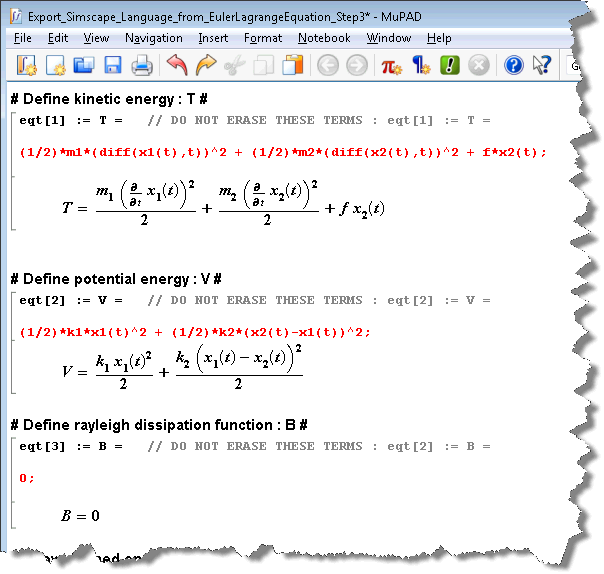The rest of the notebook implements the Euler Lagrange equation: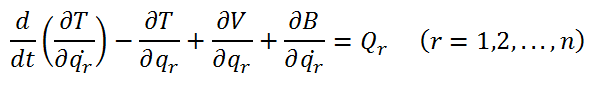So all you need to do is evaluate the notebook: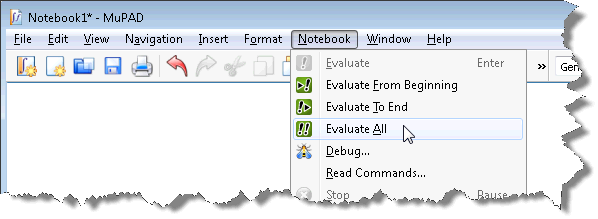and you should obtain the equations of motion of the system: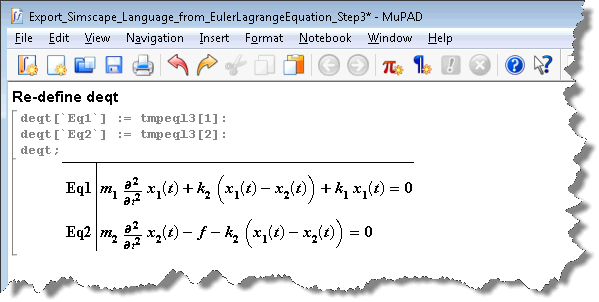Now that we have the equations of motion of the system, we want to bring them into Simulink. For that, we use the Simscape language.

In the MuPAD notebook, it is possible to generate Simscape equations using the generate function: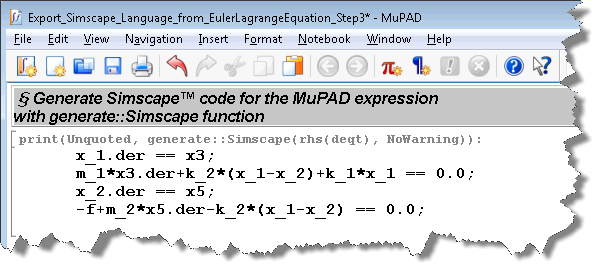We can then copy and paste this code in the equations section of a Simscape file. We let you write the rest of the Simscape so you can integrate the equations the way you want. In my case, I created a component with two ports, keeping the motion of the middle mass internal to my component. I also modified the names of a few variables auto-generated by MuPAD: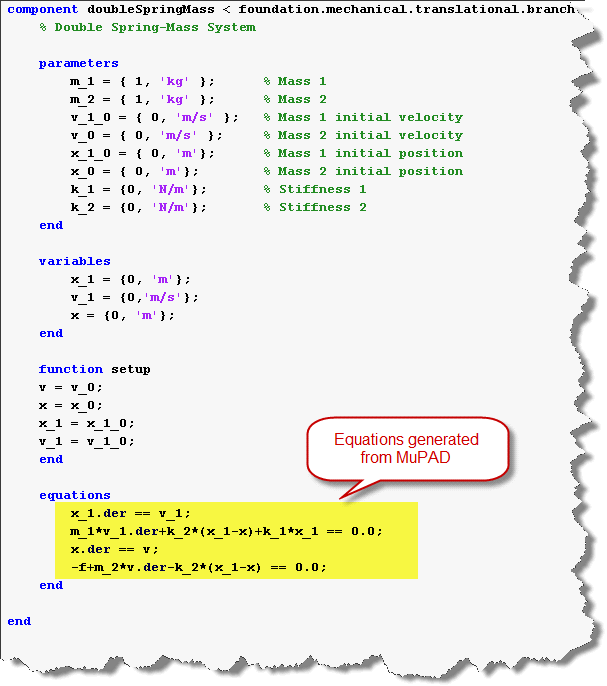I can then execute ssc_build on this file and obtain a block that I can use in Simulink: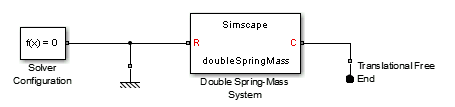Using the Simscape Simulation Results Explorer I can see that the two masses are moving as expected.